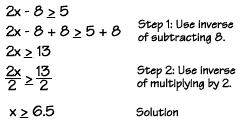Home    |    Teacher    |    Parents    |    Glossary    |    About UsNow we'll solve some more complicated equations and inequalities - ones that have two-step solutions, because they involve two operations. Remember, solving equations is like solving a puzzle. Just keep working through the steps until you get the variable you're looking for alone on one side of the equation.

Here's a two-step equation. Let's start with the variable x, and describe, step by step, what is being done to x in an equation.

 3x - 10 = 14 Equation 3x First, x is multiplied by three. 3x - 10 Next, ten is subtracted from the term 3x. 3x - 10 = 14 We get a result of 14. Start with x --> Multiply by 3 --> Subtract 10 -->  Result is 14.

Solving an equation is like running the equation backwards to discover what number will work in the equation. Now let's work backwards and use inverse operations to undo all the steps. We can start with the result of 14.

 14 Start with result. 14 + 10 Next, working backwards, we can add 10, which is the inverse of subtracting 10. 14 + 10 3 Now we divide by 3, since that's the inverse of multiplying by 3. 24 = 8 3 We get an answer of 8. Start with result of 14 —> Add 10 —> Divide by 3  Answer is 8.

Do you see how it's important when solving an equation to "undo" all the steps in the correct order? No matter how many steps are in the original equation, you can work backwards and apply the inverse operations, in order, to arrive at the solution!

You can solve two-step inequalities in exactly the same way. Just work backwards, using the inverse operations, to arrive at the solution. But watch out when multiplying or dividing by a negative number! Just as you learned in the last lesson, you must reverse the inequality symbol.Homework Help | Algebra | Equations and InequalitiesEmail this page to a friendSearch·  Solving addition and     subtraction equations·  Multiplication equations·  Division equations·  Inequalities·  Formulas·  Two-step equations     and inequalitiesFirst Glance In Depth Examples WorkoutTwo step equations and inequalities